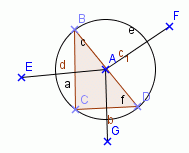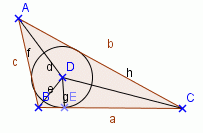Email us to get an instant 20% discount on highly effective K-12 Math & English kwizNET Programs!

#### Online Quiz (WorksheetABCD)

Questions Per Quiz = 2 4 6 8 10

### Geometry2.26 Circumcenter and Incenter

Circumcenter:
1. The perpendicular bisectors of the sides of a triangle are concurrent. The point of concurrence of the perpendicular bisectors of the sides of a triangle is equidistant from the vertices of the triangle.

2. The circle passing through the vertices of a triangle is called the circumcircle.

3. The centre of a circumcircle is called circumcenter.

Example:Identify the circumcircle, circumradius and circumcenter of the above triangle.

Solution:
Here 'c' is the circumcircle, 'AH' is the circumradius and 'A' is the in center.

5. If we draw an acute triangle and we observe that, its circumcenter falls within the triangle that is in the interior of the triangle.

6. If we draw a right triangle and we observe that its circumcenter falls on the hypotenuse of the triangle and it is midpoint of the hypotenuse.

7. If we draw an obtuse triangle and we observe that its circumcenter falls outside the triangle, that is in the exterior of the triangle.

8. The internal bisectors of the angles of a triangle are concurrent.

Incenter:
1. The point of concurrence of the internal bisectors of the angles of triangle is equidistant from the sides of the triangles.

2. The circle that touches the three sides of a triangle and lies inside the triangle is called the incircle of the triangle.

3. Its centre which is the point of concurrence of the internal bisectors of the angles of the triangle is called incentre of the triangle.

4. The radius of the incircle is called the inradius of the triangle. The incentre is equidistant from the sides of the triangle.

Example:Identify the incircle, inradius and incentre of the above triangle.

Solution:
In the above figure 'c' is the incircle, 'AE' is the inradius and 'D' is the incentre.

Review

Acute angled triangleRight angled triangleObtuse angled triangle
Circumcenter
See how the circumcenter 'A' varies in all the three types of triangles.Incentre
See the incentres 'D' of all the three types of triangles.Directions: Read the above review points carefully and answer the following questions:
1. Illustrate each of the above review points by drawing a the circumcentre and incentre of triangles.
2. Explain in your own words the circumcentre and incentre of a triangle.
3. Draw the circumcentre and incentre of the triangle with sides 10 cm, 8 cm and 6 cm. Also measure the angles.

 Q 1: The perpendicular bisectors of the sides of a triangle are ______.circumcirclescircumcentresconcurrent Q 2: The centre of the incircle, which is the point of concurrence of the internal bisectors of the angles of the triangle is the ______.incircleincentrecoincides Q 3: The ______ of a triangle is the point of concurrence of the perpendicular bisectors of the sides of the triangle.circumcentrecircumradiuscircumcircle Q 4: The circle passing through the vertices of a triangle is the ______.circumcirclecircumcentrecircumradius Q 5: The point equidistant from the sides of a triangle is the ______.incircleincentreinradius Q 6: The radius of circumcircle is the ______.circumcentrecircumcircleiircumradius Q 7: The point equidistant from the vertices of triangle is the ______.incentreinradiuscircumcentre Q 8: In an acute angled triangle the circumcentre falls in the ______ of the triangle.interiorexteriormidpoint Question 9: This question is available to subscribers only! Question 10: This question is available to subscribers only!